# Parallel and Series Led Resistor Calculator

Series, Parallel resistor calculations to run LEDs – Led Calculator

Calculation of the resistor value in series with the LED, parallel to the LED to operate the LED at high voltages. If you do not know clearly the current drawn by the LED in the LED calculation table, enter the value as 10 mA. The standard LED voltage information is given below. In general, the outer plastic covers of Ultra bright LEDs are white and transparent. The operating voltage of these LEDs is higher than the normal colors, for example, while the normal red LED is 2V, the ultra bright red LED works with 3V, generally drawing 10..20ma current.

One of the outstanding features of the LED resistance calculation tool is the calculated LED resistance value is given as full and rounded, for example, 1k-ohm is recommended for 980-ohm, the same applies to the resistance power.

 RED 1.8V..2V ORANGE 1.9..2.2V YELLOW 1.9..2.2V GREEN 2V..3.1V BLUE 3V..3.7V WHITE 3V..3.4V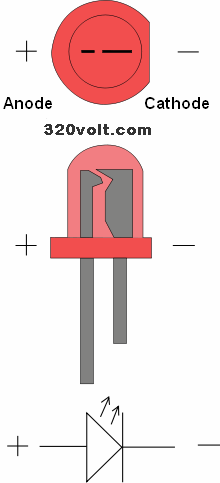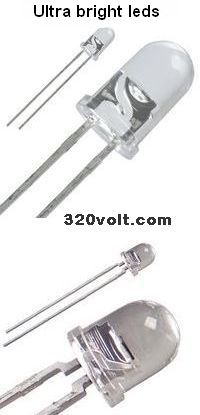### Single Led Series Resistor CalculationSupply Voltage (DC)
V
Working voltage of LED
V
Current drawn by the LED
ma

Calculated resistor value:
Ω
Calculated resistor power value:
W
Recommended resistor value:

Recommended resistance power:
W

### Multiple Led Series Resistor Calculation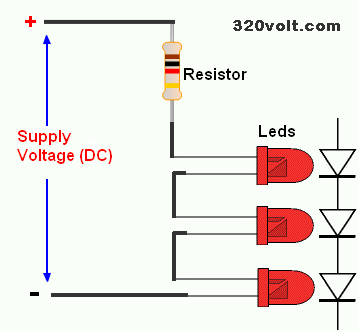Supply Voltage (DC)
V
Working voltage of LED
V
Current drawn by the LED
ma
Number of LEDs Connected in Series

Calculated resistor value:
Ω
Calculated resistor power value:
W
Recommended resistor value:

Recommended resistance power:
W

### Parallel Led Resistor Calculation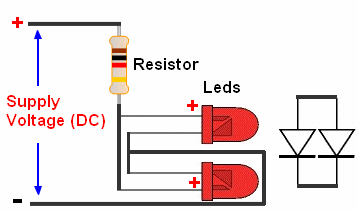Supply Voltage (DC)
V
Working voltage of LED
V
Current drawn by the LED
ma
Number of Leds Connected in Parallel

Calculated resistor value:
Ω
Calculated resistor power value:
W
Recommended resistor value:

Recommended resistance power:
W

Even if the number of LEDs is determined in the LED calculations, the example diagram will give an idea about the resistor connection of more than one LED with different connection types for those who are new to electronics or have no experience.

In the circuit diagram, there are 4 different connection types to operate 15 LEDs with 12v. By connecting the LEDs in parallel, it will be easier with a single resistor, but with this method, the life of the LEDs will be very short because even if the brand and model of the LEDs are the same, the internal resistances are different, and you have to know the working voltage of the LED and the current it draws.

For example; You want to connect 12v and 10 leds in parallel and use it. In parallel led calculation, you entered the led current of 20ma. You will connect the operating voltage of 3v 10 leds in parallel. The result of the calculation is 45 ohms, but if the current drawn by the leds is 10ma, the resistance value is 100 ohms. If you use a low value resistor because you entered it incorrectly, the life of the leds will be even less. You will not have this problem in serial connection.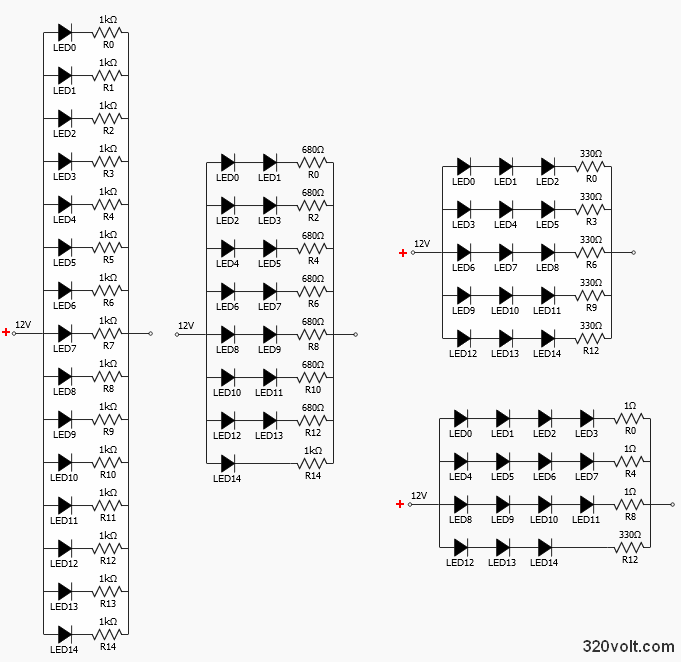### LED Resistor Calculation FormulaFirst, let's calculate the resistor value, for this we need to know the Power supply voltage, the operating voltage of the LED and the current consumption of the LED.

For example, our power supply is 12 volts, LED voltage is 3.4 volts and current is 15Ma calculation; 12 – minus 3.4 = 8.6 we divide the result by the LED current 8.6 / .015 = 573 so the result is 573-ohm

It will be difficult to find a 573-ohm resistor. In this case, slightly higher values ​​can be used, such as 620….680-ohms..

Calculating Power of LED Resistor

We do a similar process to calculate the power of the resistor to be connected to the LED 12 – minus 3.4 = 8.6 we multiply the result with the LED current 8.6 x .015 = 0.129 in this case we can use the result as 0.129W standard 1/4W resistor 0.250W.

The power of the resistor can be used more than the calculated value. For example, in the calculation, a suitable value is found for the 1/4w resistor, but you have a 1w or 5w resistor, it can be used at these values.

Serial, Parallel Connection Calculations

How will the serial LED be calculated? When we connect the LEDs in series, the operating voltage increases. For example; If you connect 3 LEDs working with 2 volts in series, the operating voltage will be 6 volts, accordingly the calculation will be made 12 – minus 6 = 6

In Parallel LED Connection; The current will increase. When you connect 3 15ma LEDs in parallel, the total LED current will be 45mA, and the calculation will be made accordingly.

In a parallel LED connection, the current is not shared equally because of the manufacturing tolerances on the forward voltages of the LEDs. Running two or more LEDs in parallel from a single current source can cause LED failure over time. If possible, it would be healthier to add a separate resistor for each LED in parallel LED connection.